## Introduction

We can create and solve number puzzles and games. There are many interesting puzzles in maths. Watch this video to play the game.

In the video above, we played a game where we did the following:

• Take any two-digit number
• Find the sum of the digits
• Subtract the sum from the number that you chose

Try the same with a different number. Did you get the same result? Is your answer being always a multiple of 9?

Why does this happen? Let us understand the math behind this magic.

• Let the number that you thought initially be 10x + y

where x is tens digit and y is ones digit

• On finding sum of the digits we get

x + y

• If we subtract the sum from the original number, we get

10x + y – ( x + y) = 10x + y – x – y = 9x

Whatever be the original number, the result will always be multiple of 9.

In mathematics, there are various types of numbers. Also, we have studied about interesting properties about them.

There is a good chance that you are familiar with the Rubik’s cube: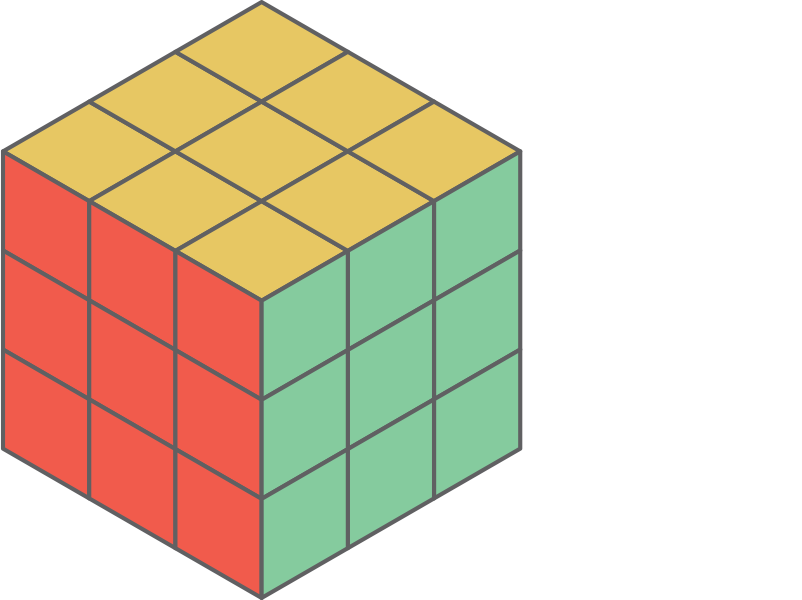Have you ever tried solving one?

The cube is a 3 × 3 × 3 arrangement of smaller cubes with six faces of the large cube. These six faces of the cube can be rotated so that the scrambled cube can be solved in such a way that each face has a single colour of cubes.

In total there are 43,252,003,274,489,856,000 different arrangements of the small cubes!

To put the number in perspective, if you had a cube for every one of these arrangements, then you could cover the surface of the Earth (including the oceans) about 250 times!

#### Concepts

The chapter ‘Playing With Numbers’ covers the following concepts:

#### Numbers in the General Form

We know how to expand numbers by placing them in the place value table. This is done by multiplying each digit by its place value and then adding them together.

For examples:

• Expanded form of 46 = 4 × 10 + 6 × 1

i.e., 46 = 4 × 10 + 6

• Expanded form of 53 = 5 × 10 + 3 × 1

i.e., 53 = 5 × 10 + 3

Is there any pattern in these expanded forms of digits? We expanded the two-digit numbers ‘ab’ and ‘ba,’ i.e.,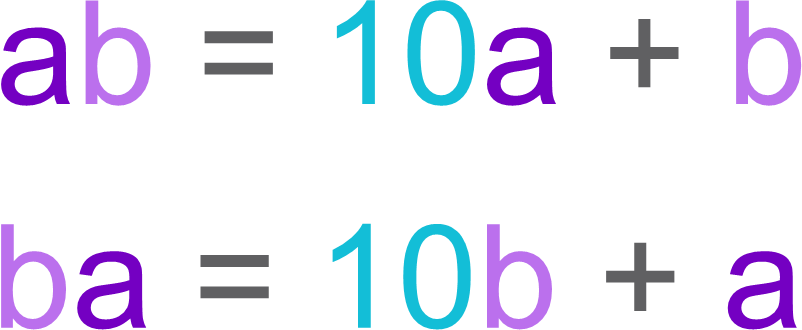This form of numbers is called as generalised form of number. Generalised form of numbers is obtained by multiplying each digit by its place value and adding them together.

To three-digit numbers ‘abc’: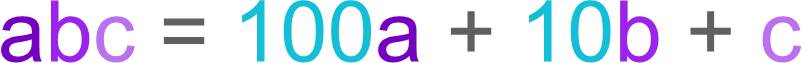Similarly,

• The generalised form of the number ‘bca’ is 100b + 10c + a
• The generalised form of the number ‘cab’ is 100c + 10a + b

Let us take the generalised form of number 39, i.e., 39 = 10 × 3 + 9

The usual form of 10 × 3 + 9 is 39.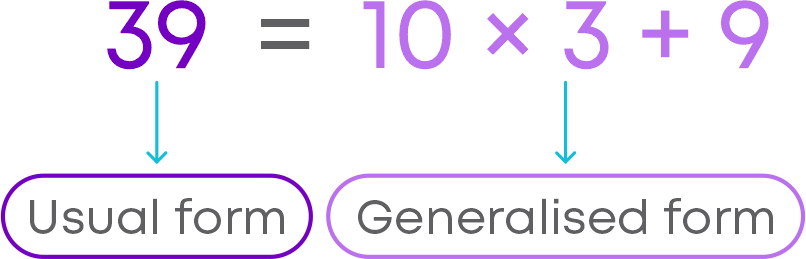Usual form is obtained by simplifying the generalised form.

#### Reverse the Digits of Two- and Three- Digit Numbers

Arun and Rohan are playing with numbers by reversing the digits of the number. Arun asked Rohan to take any two-digit number. Rohan took 32.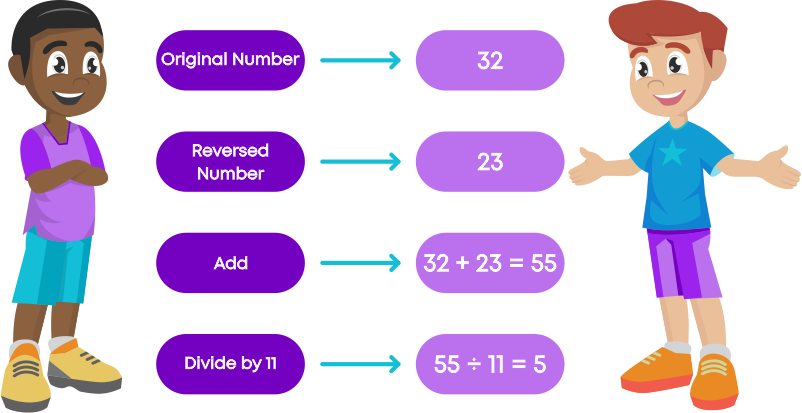Arun predicted that there would be no remainder and Rohan got the answer 5 with no remainder. How did he predict that there will be no remainder?

What is the trick behind this game?

• We know that the general form of two-digit number ab is 10a + b.

On reversing the digits, we get 10b + a. Now, add them.

i.e., 10a + b + 10b + a = 11a + 11b = 11 (a + b)

• We can see here, the sum of a two-digit number and its reverse is always the multiple of 11.

Arun knows this trick so he predicted that there will be no remainder.

• Also, observe that if we divide the sum by 11, the quotient is (a + b), which is exactly the sum of digits chosen from ab.

Now, Arun and Rohan are playing another game. Arun asked Rohan to take any two-digit number. Rohan took 67.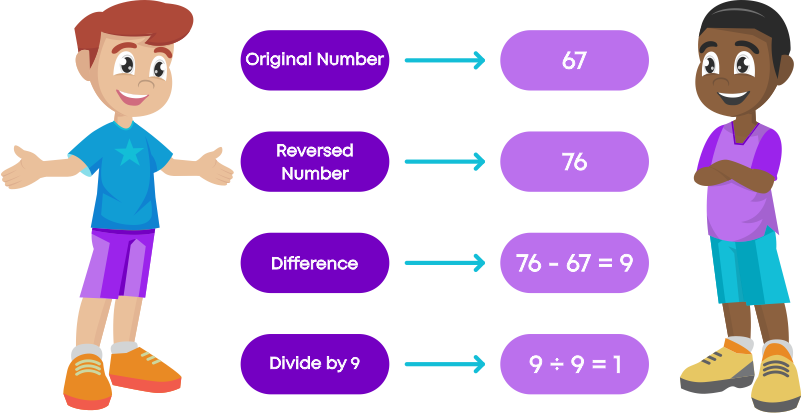Rohan got the answer as 1 and there was no remainder. This time, Rohan explained the trick of this game.

What is the trick behind the game?

• You know that the general form of two-digit number ab is 10a + b.

On reversing the digits, we get 10b + a. Now, find the difference between two numbers

i.e., 10a + b – (10b + a) = 9a - 9b = 9 (a - b)

• We can see here, the difference of a two-digit number and its reverse is the multiple of 9.

Rohan knows this trick so he predicted that there will be no remainder.

• Observe that if we divide the difference by 9, the quotient is (a - b), which is exactly the difference between digits chosen from ab.

Also, note that we need to subtract the smaller number from bigger number.

Now, Arun asked Rohan to take a three-digit number. Rohan took 379. Then, Rohan asked Arun if there was any pattern in reversing a three-digit number.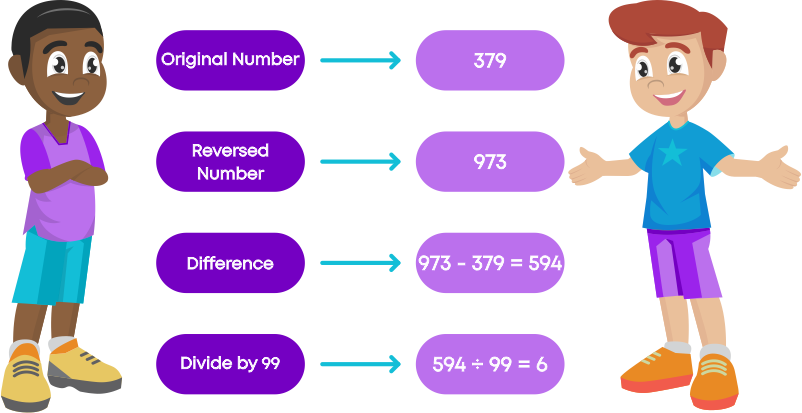Arun predicted that there would be no remainder and Rohan got the quotient as 6. How did he predict that there would be no remainder?

What is the trick behind the game?

• You know that the general form of three-digit number abc is 100a + 10b + c.

On reversing the digits, we get cba.

i.e., 100c + 10b + a

Now, find the difference of two numbers.

• If a > c, then the difference between the numbers is

(100a + 10b + c) – (100c + 10b + a)

= 100a + 10b + c – 100c – 10b – a

= 99a – 99c

= 99(a – c)

• If c > a, then the difference between the numbers is

(100c + 10b + a) – (100a + 10b + c)

= 99c – 99a

= 99(c – a)

And, of course, if a = c, the difference is 0.

• We can see here, the difference of a three-digit number and its reverse is a multiple of 99 in each case.

Arun knows this trick so he predicted that there would be no remainder.

Observe that if we divide the difference by 99, the quotient is (a - c) or (c - a).

Note that we need to subtract the smaller number from bigger number.

Remember these properties:

Property 1: the sum of a two-digit number and its reverse is always a multiple of 11.

Property 2: the difference of a two-digit number and its reverse is always a multiple of 9.

Property 3: the difference of a three-digit number and its reverse is always a multiple of 99.

Consider the three-digit number 429. Now, let us form another two numbers using the digits 4, 2 and 9. Take the first number by shifting one’s digit to the left end.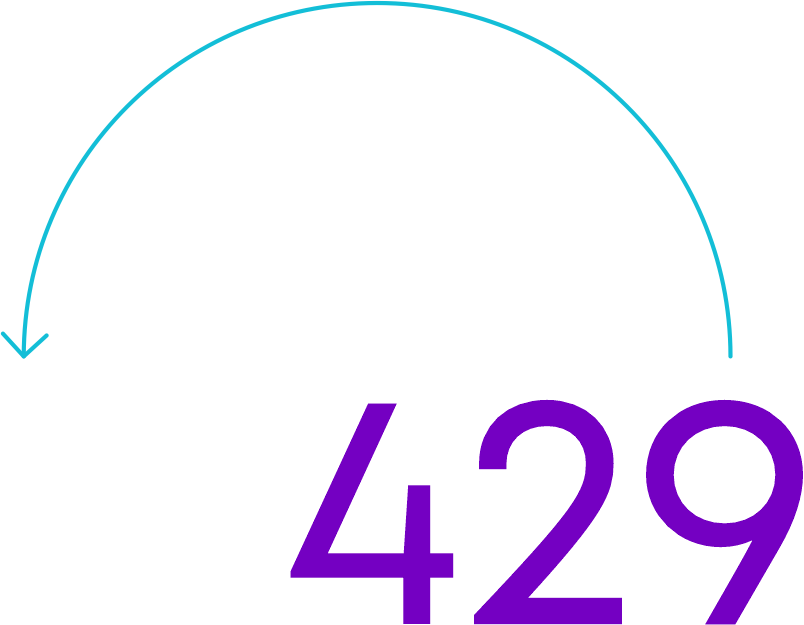i.e., 942

Take the second number by shifting hundreds digit to the right end.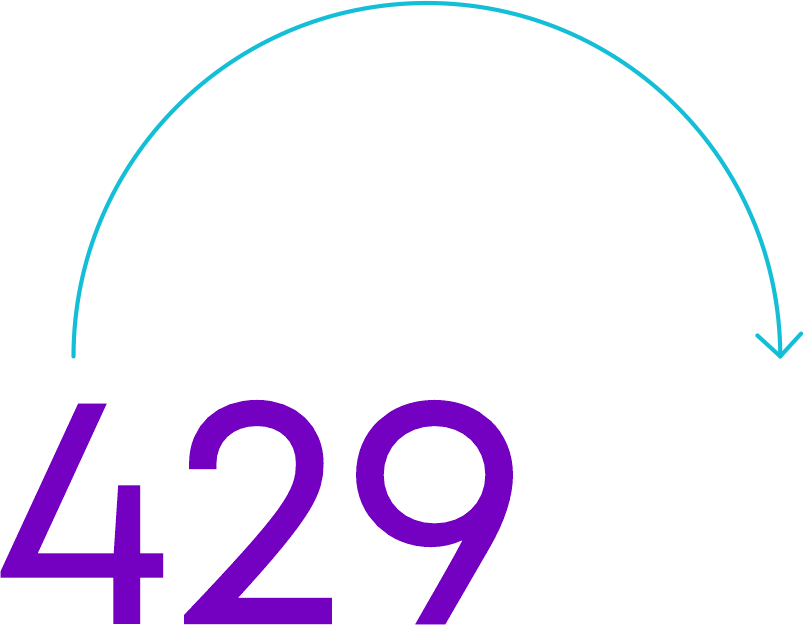i.e., 294

Sum of 429, 942, and 294 is 429 + 942 + 294 = 1665

Now, let us take the general form of three-digit number abc, i.e., 100a + 10b + c.

• We can take other two numbers as cab and bca.

i.e., 100c + 10a + b and 100b + 10c + a

• Now, let us add the three numbers.

abc + cab + bca

= 100a + 10b + c + 100c + 10a + b + 100b + 10c + a

= 111a + 111b + 111c

= 111 (a + b + c)

= 37 × 3 (a + b + c)

From this we can see that their sum is always divisible by 37.

Property: the sum of three-digit numbers, abc, bca, and cab is always divisible by 37. If we divide the sum by 37, then the quotient obtained is 3(a + b + c).

Tanu was solving a problem to find the value of letters. The problem is given below.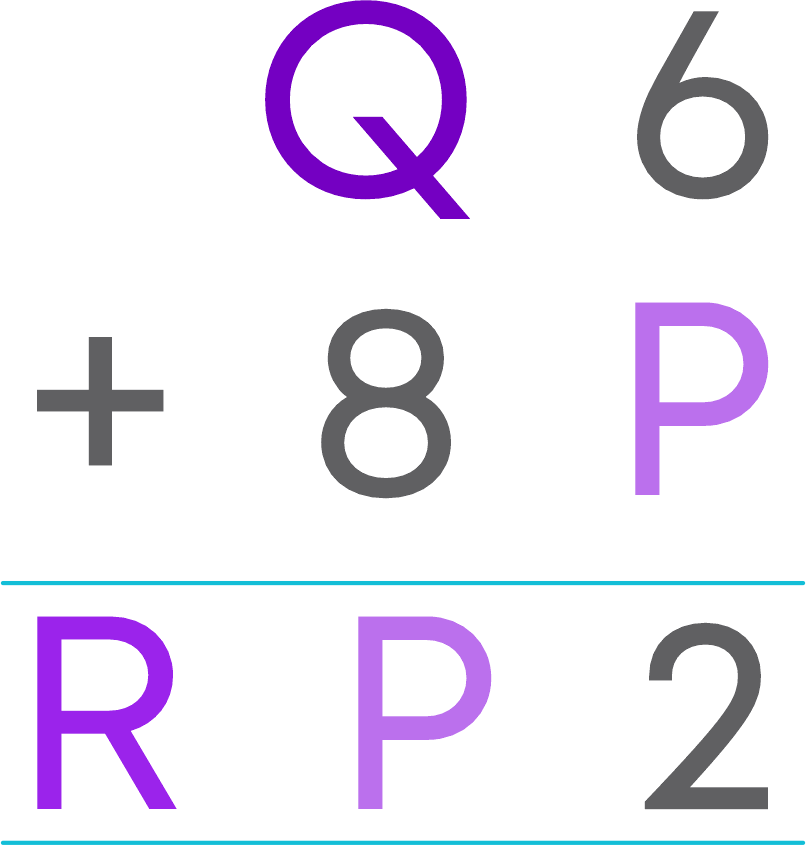From this, we can see that the addition of 6 and P gives a number whose ones digit is 2, i.e., 6 + P = 2. Therefore, the possible value of P is 6. If P = 6 then 6 + 6 = 12, 1 will be carried forward in the next step.

Now, RP = Q + 8 + 1

R6 = Q + 9

i.e., Q + 9 is a number whose ones digit is 6.

Hence, Q = 7 and R = 1.

So, the values of P, Q, and R are 6, 7, and 1, respectively.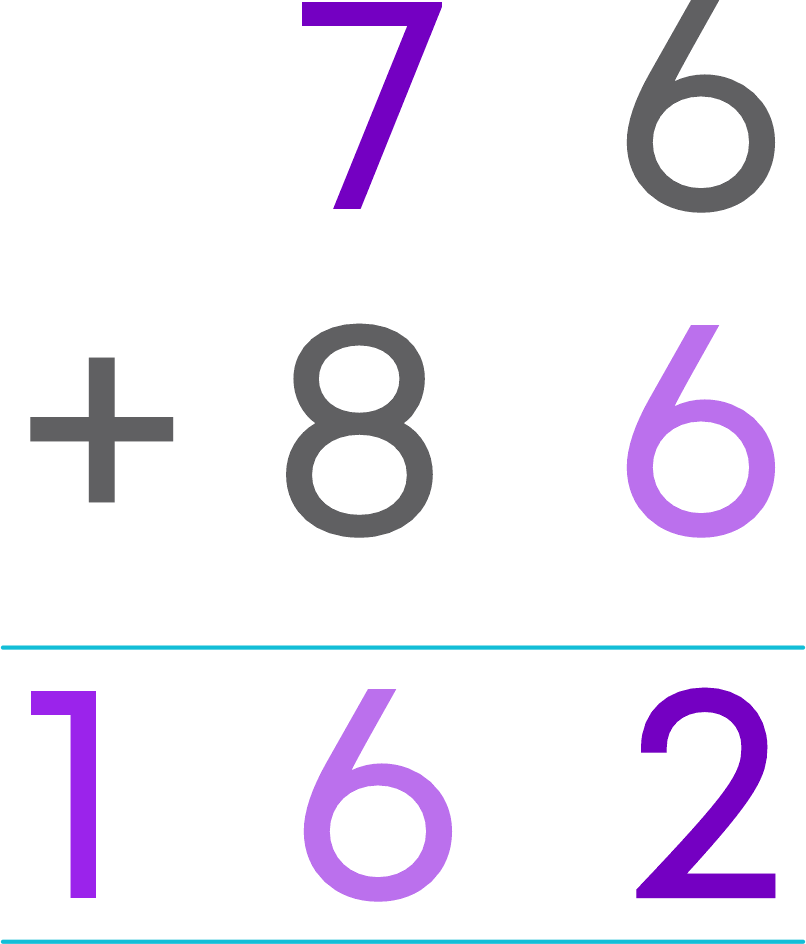We know how to find the value of letters when the operation involved is addition. Now, Sanvi was solving problem to find the value of letters which involves the operation of multiplication. The problem is given below.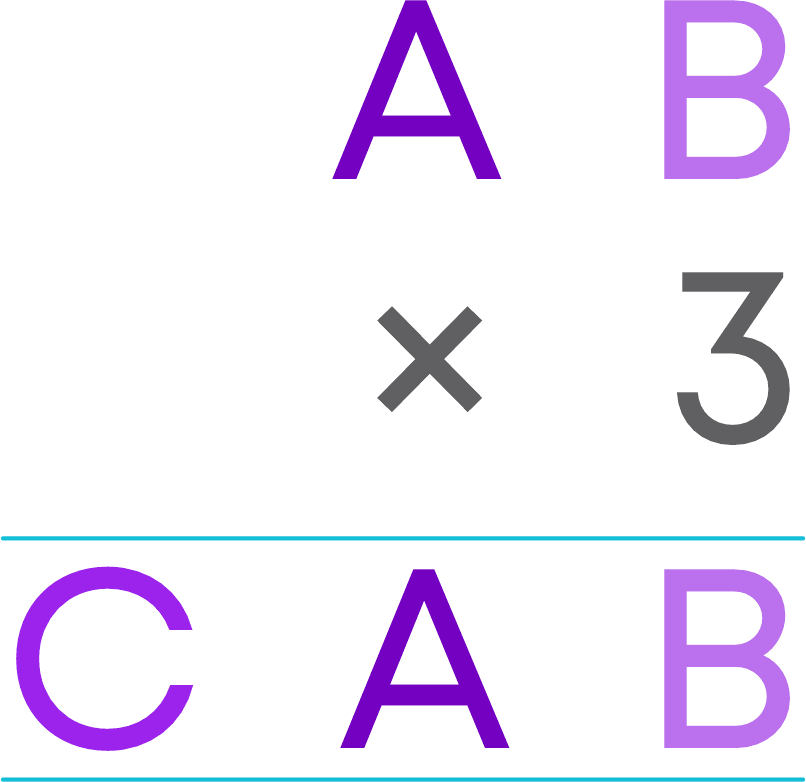From this, we can see that the multiplication of B and 3 gives a number whose ones digit is B again. Hence, B must be 0 or 5.

Case 1: B = 5

First step of multiplication gives 3 × 5 = 15

1 will be carried forward in the next step.

In the next step, we get 3 × A + 1 = CA

This is not possible for any values of A.

Hence, B must be 0 only.

Case 2: B = 0

If B = 0 then 0 × 3 = 0 there will be no carrying for the next step.

In the next step, 3 × A = CA

i.e., one’s digit of 3 × A should be A. So, A should be 0 or 5.

We cannot take A as 0, since AB is a two-digit number.

Hence, A = 5.

So, we obtain the multiplication as given below.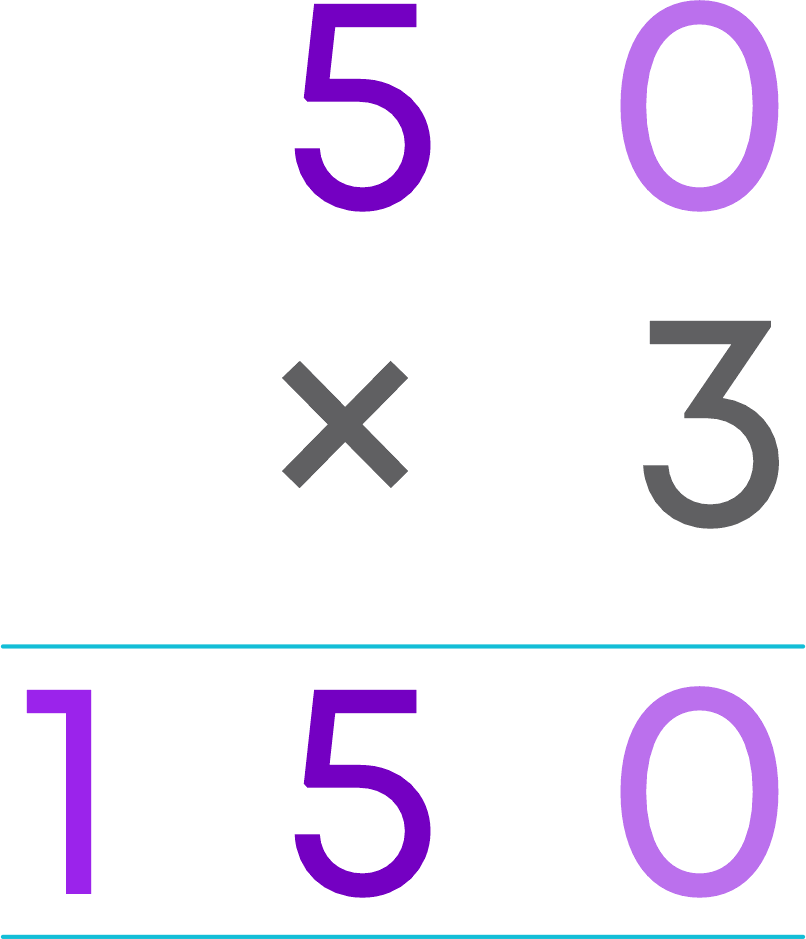Hence, A = 5, B = 0, and C = 1.

#### Divisibility Rules

A number is divisible by 10 if its ones digit is 0. In other words, if the ones digit of a number is ‘0’ then the number is multiple of 10 and if the ones digit of a number is not ‘0’ then the number is not multiple of 10.

Now, let us see the reason for the divisibility of numbers by 10 when numbers are written in general form. Suppose the number is cba.

We can write this number in general form as 100c + 10b + a

Clearly, ‘a’ is at ones place, ‘b’ is at tens place and ‘c’ is at hundreds place. We know that 10, 100 are divisible by 10. So 10b, 100c are also divisible by 10. To say the number is divisible by 10, ‘a’ should be divisible by 10. What is the possible value of ‘a’ so that it should be divisible by 10?

he only possible value of ‘a’ is 0.

This is the reason behind the divisibility test for 10.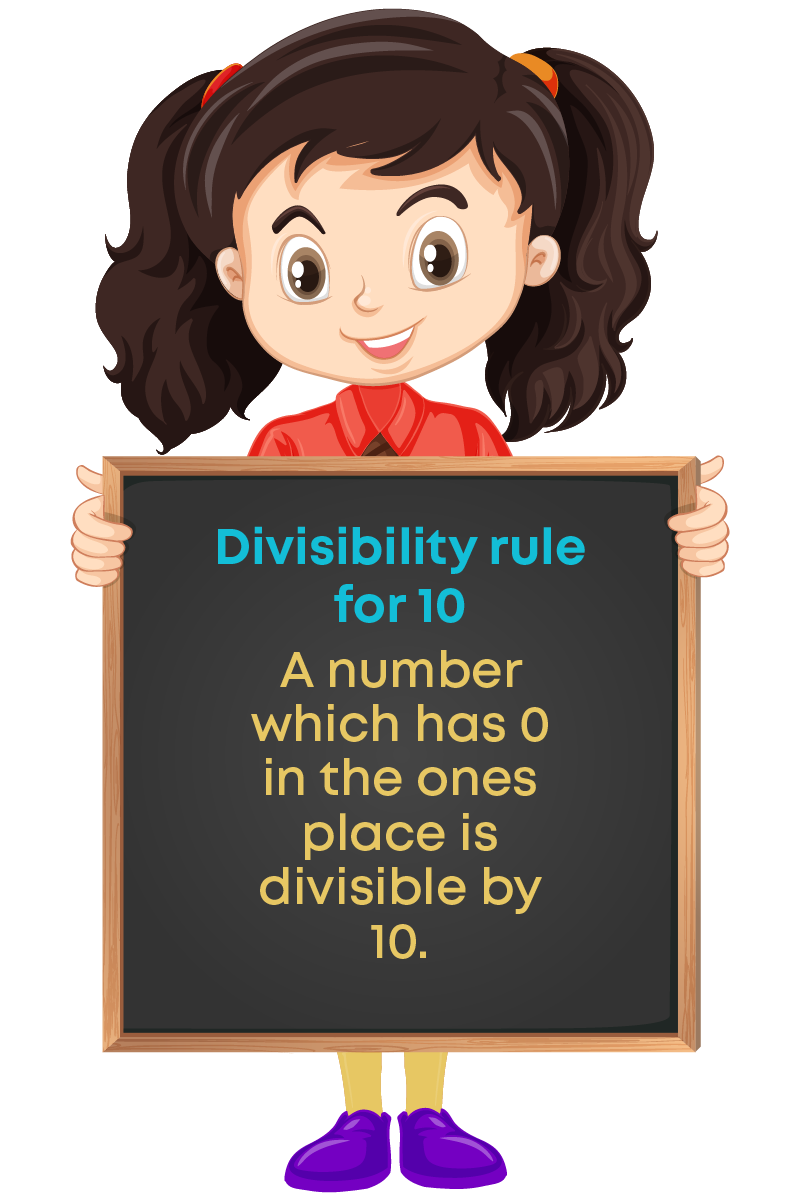A number is divisible by 5 if its ones digit is 0 or 5. In other words,

• If the ones digit of a number is ‘0’ or 5 then the number is a multiple of 5.
• If the ones digit of a number is other than ‘0’ or 5 then the number is not a multiple of 5.

Now, let us see the reason for the divisibility of numbers by 5 when numbers are written in general form. Suppose the number is cba. We can write this number in general form as 100c + 10b + a. Clearly, ‘a’ is at ones place, ‘b’ is at tens place and ‘c’ is at hundreds place.

We know that 10, 100 are divisible by 5 since they are multiple of 5. So 10b, 100c are also divisible by 5.

To say the number is divisible by 5, ‘a’ should be divisible by 5. What is the possible value of ‘a’ so that it should be divisible by 5?

The possible value of ‘a’ is either ‘0’ or 5.

This is the reason behind the divisibility test for 5.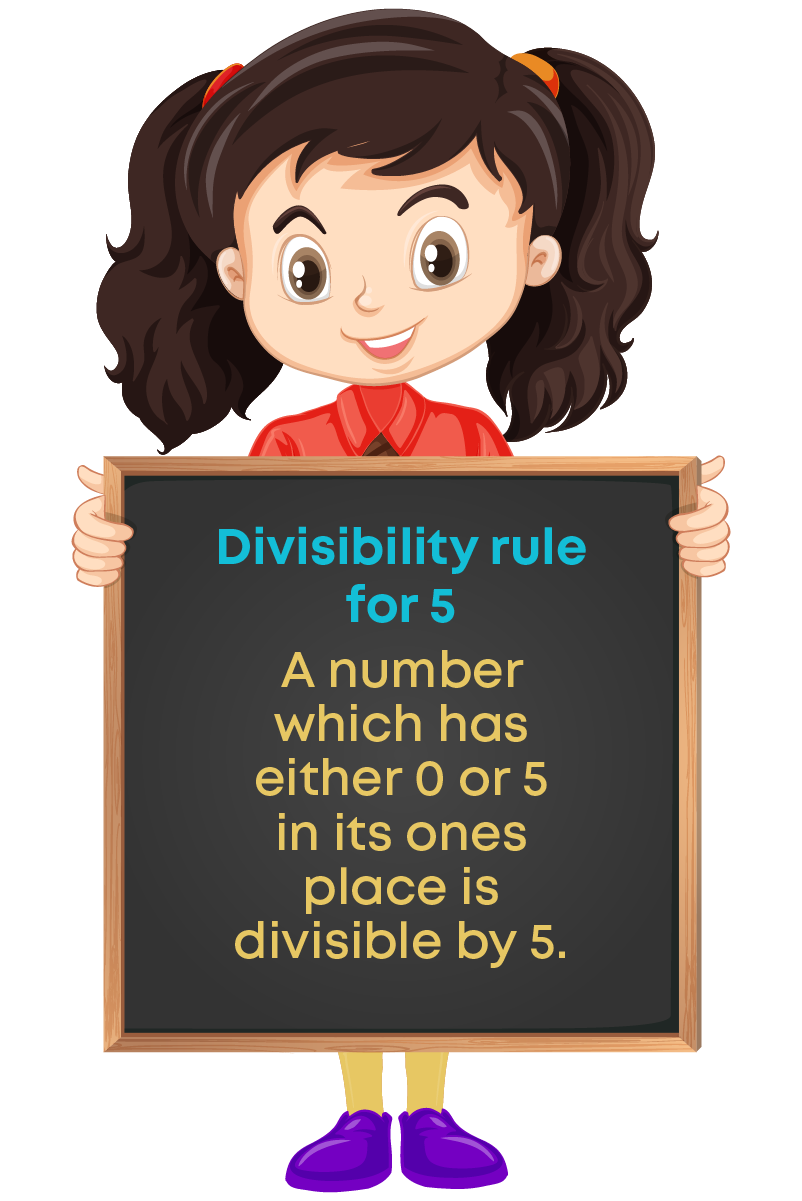A number is divisible by 2 if it has any of the digits 2, 4, 6, 8 or 0 in its ones place. In other words, if the ones digit of a number is 2, 4, 6, 8 or 0 then they are even numbers. All even numbers are multiples of 2.

Now, let us see the reason for the divisibility of numbers by 2 when numbers are written in general form. Suppose the number is cba. We can write this number in general form as 100c + 10b + a. Clearly, ‘a’ is at ones place, ‘b’ is at tens place and ‘c’ is at hundreds place.

We know that 10, 100 are divisible by 2 since they are multiples of 2. So 10b, 100c are also divisible by 2. To say the number is divisible by 2, the ones digit ‘a’ should be divisible by 2.

What is the possible value of ‘a’ so that it should be divisible by 2? The possible value of ‘a’ is 2, 4, 6, 8 or 0.

This is the reason behind the divisibility test for 2.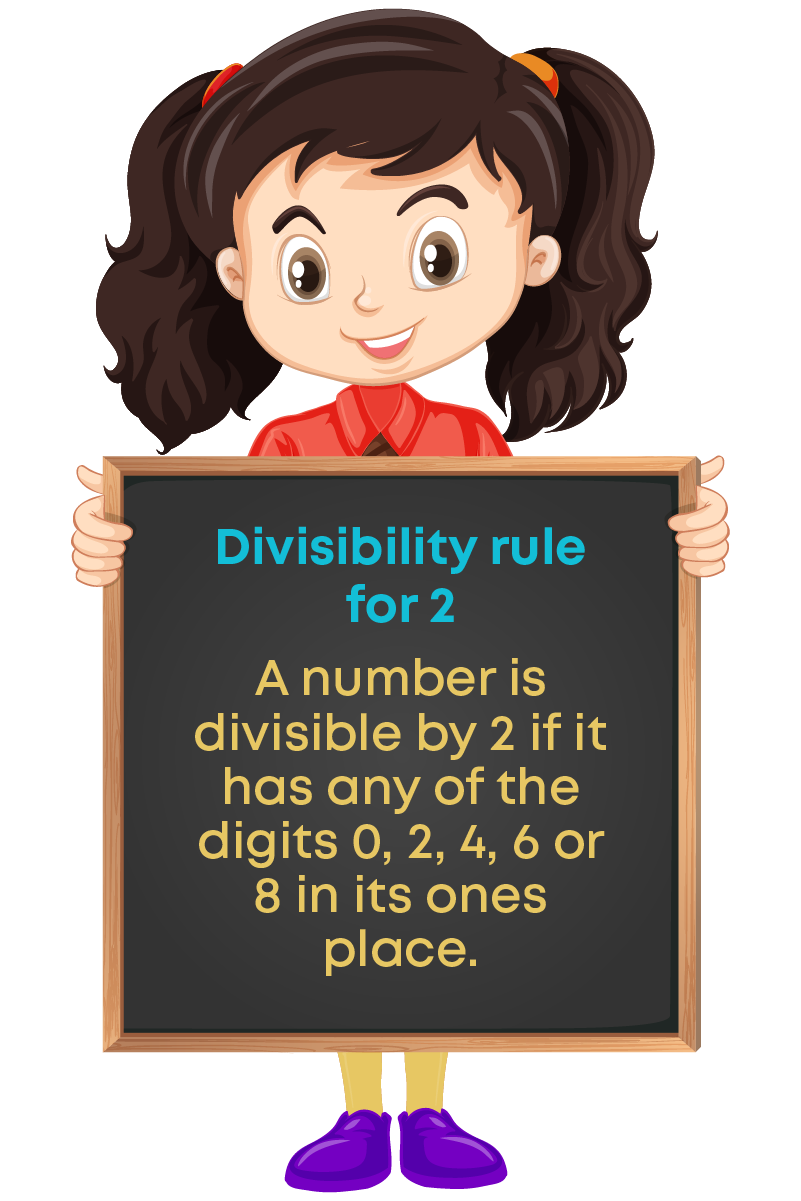Divisibility by 3:

• A number is divisible by 3 if the sum of the digits is divisible by 3

Divisibility by 9:

• A number is divisible by 9 if the sum of the digits is divisible by 9.

We saw that the divisibility rules for 2, 5 and 10 by writing the numbers in general form. Now, let us see the reason for the divisibility of numbers by 3 and 9 when numbers are written in general form. Suppose the number is cba. We can write this number in general form as 100c + 10b + a

i.e., 100c + 10b + a

= 99c + c + 9b + b + a

= 9 (b + 11c) + (a + b + c)

To say the number is divisible by 9 (or 3), the sum of the digits should be divisible by 9 (or 3). Clearly, the first term 9(b + 11c) is divisible by 9. Also, 9(b + 11c) is divisible by 3. Then what will you say about the rule for divisibility of 3 and 9?

We can say the number 100c + 10b + a is divisible by 9 (or 3) if a + b + c is divisible by 9(or 3).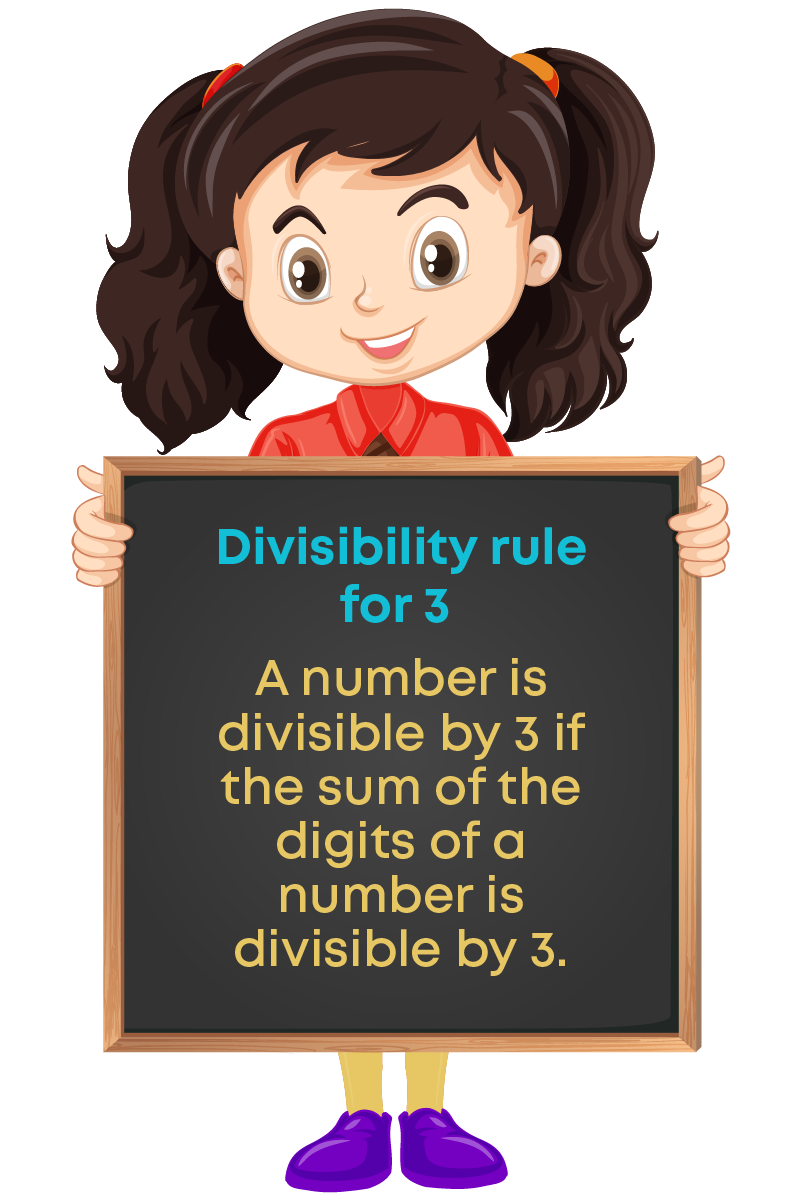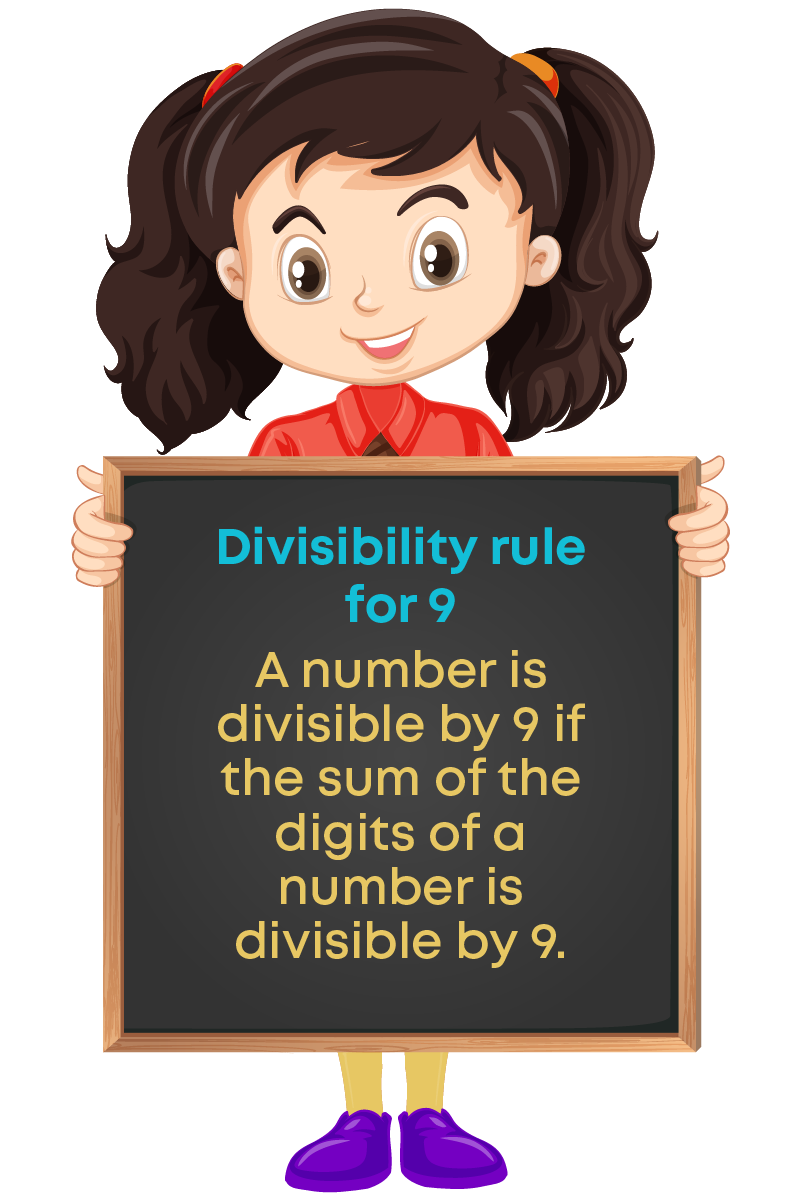There are some questions in which we know that the given number is divisible by 3 or 9, but we need to find the unknown digit in the number. Consider the question.

If 1y16 is a multiple of 9, where y is a digit then what is the value of y?

Given that, 1y16 is a multiple of 9 and y is a digit. We know that if a number is a multiple of 9, then the sum of the digits will be divisible by 9.

Sum of digits of 1y16 = 1 + y + 1 + 6 = 8 + y

So, 8 + y should be multiple of 9.

This is possible when 8 + y is any of the numbers 0, 9, 18, 27 and so on.

i.e., 8 + y = 0, 9, 18,,,,,

• If 8 + y = 0, then y = (-8)
• If 8 + y = 9, then y = 1
• If 8 + y = 18 then y = 10

Since y is a single-digit number, 8 + y can only be 9.

Therefore, y should be 1 and the number is 1116.

#### Common Errors

The following are topics in which students make common mistakes when dealing with numbers:

• 1. Confusion between odd and prime numbers
• 2. Confusion in writing the generalized form of a number
• 3. The sum of the three 3-digit numbers 429, 942 and 249 using digits 2,4 and 9 is not divisible by 37

#### Confusion Between Odd And Prime Numbers

Which of the following are prime numbers?

2, 3, 4, 5, 7, 9, 13, 16.

The prime numbers are 2, 3, 5, 7 and 13. Many times, the terms ‘prime number’ and ‘odd number’ may cause confusion. We know that 2 is the only even prime number and all other prime numbers are odd. That does not mean that all the odd numbers are prime numbers.

is not a prime number since it has more than 2 factors, i.e., 1, 3 and 9. For better understanding let us categorise the numbers as follows.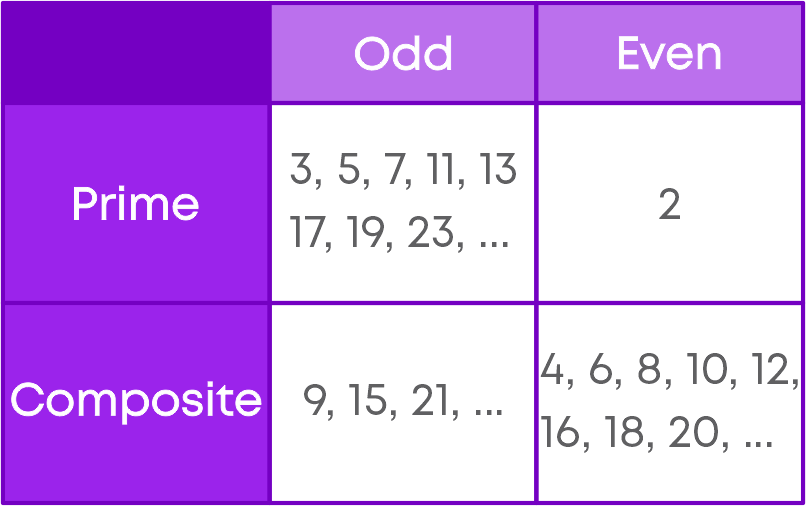#### Confusion In Writing The Generalized Form Of A Number

A two-digit number represented by ‘ab’ is not a × b.

It can be written as ab = 10a + b.

So, the generalized form of 63 is 10 × 6 + 3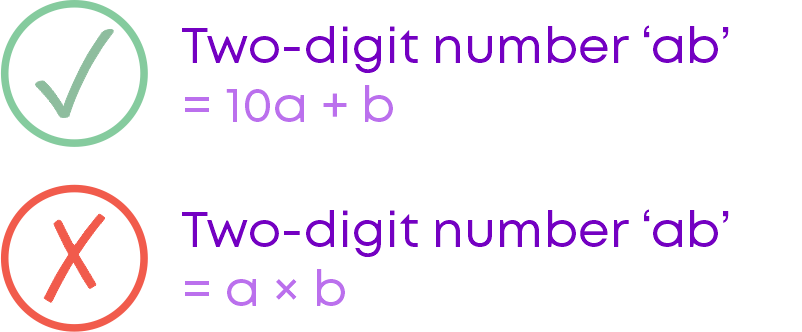A three-digit number represented by ‘abc’ is not a × b × c.

It can be written as abc = 100a + 10b + c.

So, the generalized form of 244 is 100 × 2 + 10 × 4 + 4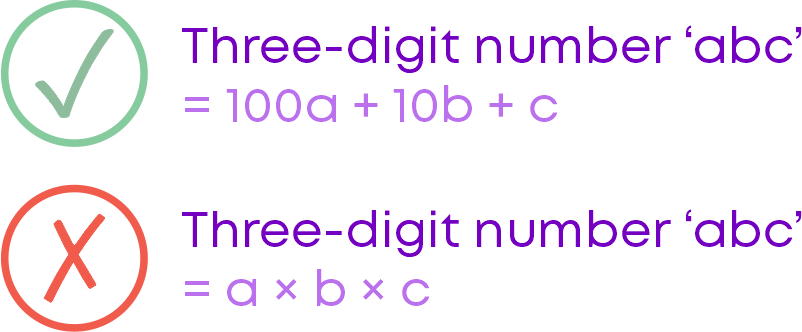#### The Sum Of The Three 3-Digit Numbers 429, 942 And 249 Using Digits 2,4 And 9 Is Not Divisible By 37

When we form the three-digit numbers using the given three digits 4, 2 and 9, their sum will always be a multiple of 37.

But while making three-digit numbers using the given three digits, be careful and follow this rule:

Take the first number by shifting one’s digit to the left end.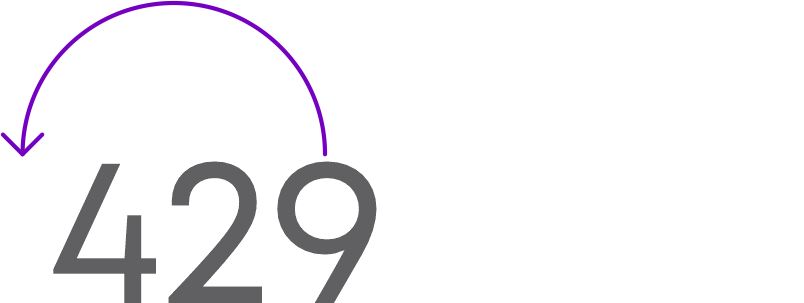i.e., 942

Take the second number by shifting the hundreds digit to the right end.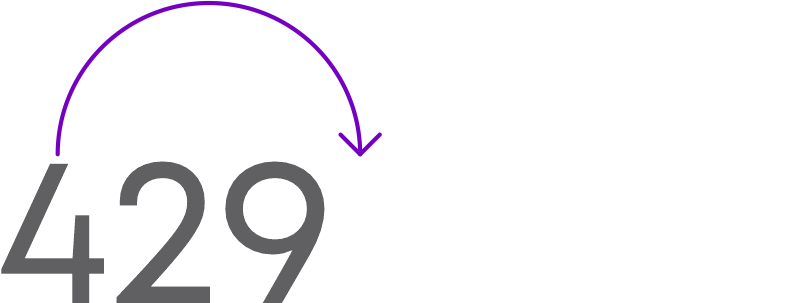i.e., 294

Sum of 429, 942 and 294 is 429 + 942 + 294 = 1665 which is divisible by 37.

But the sum of the randomly formed numbers 429, 942 and 249 is 429 + 942 + 249 = 1620, which is not divisible by 37.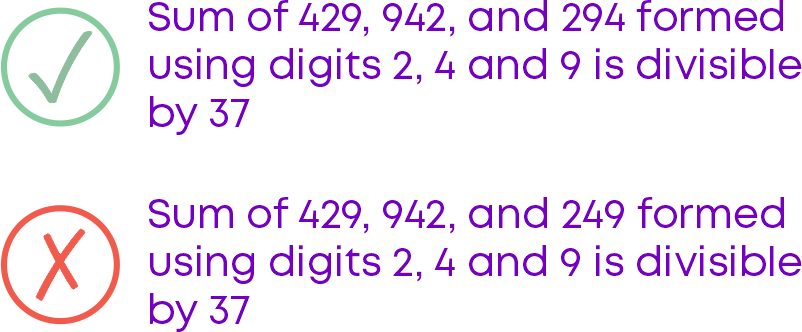#### Conclusion

Now that you have learnt about numbers and their properties, can you solve this riddle?

The ages of a father and son add up to 66.

The father’s age is the son’s age reversed.

The son’s age is a multiple of 5.

How old could they both be?